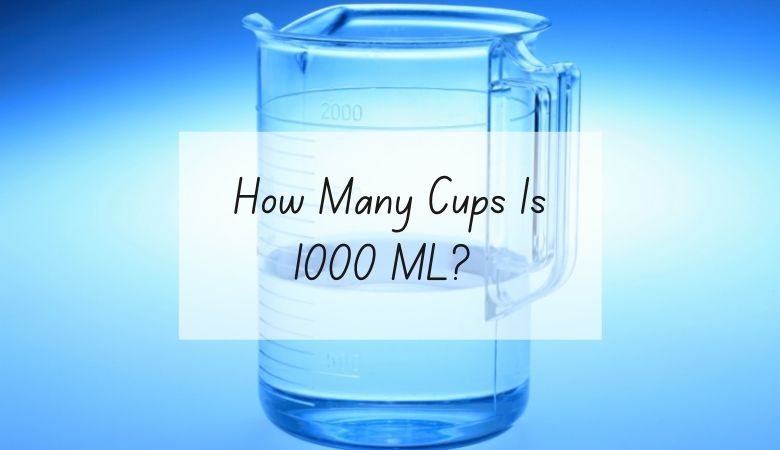# How Many Cups Is 1000 ML?

There are 4.22 cups in 1000 milliliters.

One cup and 1000 milliliters are both common measurements you might encounter, particularly if you’re baking or cooking.

Calculating how many cups are in 1000 milliliters can at first seem a bit tricky since these units are parts of two different measurement systems.

However, with a bit of simple math, you can easily calculate between cups and milliliters, and figuring out how many cups are in 1000 milliliters isn’t too difficult at all.

There are about 236.6 milliliters in a single cup.

In order to figure out how many cups are in a certain number of milliliters, then, all you would need to do is divide the number of milliliters by 236.6.

You can also round up to 237.

To figure out how many cups are in 1000 milliliters, divide 1000 by 236.6.

1000 / 236.6 = 4.22

The answer, which is 4.22, tells you that there are just over 4 cups in 1000 milliliters.

You can also reverse the math to figure out how many milliliters are in a certain number of cups.

For example, if you want to know how many milliliters are in 6 cups, simply multiply 236.6 by four.

The answer, which is 1419.6, tells you that there are just over 1400 milliliters in 6 cups.

Here is a conversion table for you to reference.

## Milliliters to cups conversion table

Recipes or other tasks that call for cup measurements are more common in the United States since this unit is a measurement in the imperial system.

Milliliters, on the other hand, are part of the metric system, which is widely used around the world.